Courses

# NCERT Solutions(Part- 2)- Cubes and Cube Roots Class 8 Notes | EduRev

## Mathematics (Maths) Class 8

Created by: Full Circle

## Class 8 : NCERT Solutions(Part- 2)- Cubes and Cube Roots Class 8 Notes | EduRev

The document NCERT Solutions(Part- 2)- Cubes and Cube Roots Class 8 Notes | EduRev is a part of the Class 8 Course Mathematics (Maths) Class 8.
All you need of Class 8 at this link: Class 8

Exercise 7.1

Question 1. Which of the following numbers are not perfect cubes?

(i) 216 (ii) 128 (iii) 1000
(iv) 100 (v) 46656

Solution:

(i) We have 216 = 2 * 2 * 2 * 3 * 3 * 3

Grouping the prime factors of 216 into triples, no factor is left over.

∴ 216 is a perfect cube.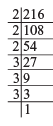(ii) We have 128 = 2 * 2 * 2 * 2 * 2 * 2 * 2

Grouping the prime factors of 128 into triples, we are left over with 2 as ungrouped factor.

∴ 128 is not a perfect cube.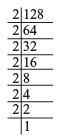(iii) We have 1000 = 2 * 2 * 2 * 5 * 5 * 5

Grouping the prime factors of 1000 into triples, we are not left over with any factor.

∴ 1000 is a perfect cube.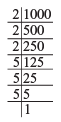(iv) We have 100 = 2 * 2 * 5 * 5

Grouping the prime factors into triples, we do not get any triples. Factors 2 * 2 and 5 * 5 are not in triples.

∴ 100 is not a perfect cube.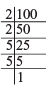(v) We have 46656 = 2 * 2 * 2 * 2 * 2 * 2 * 3 * 3 * 3 * 3 * 3 * 3

Grouping the prime factors of 46656 in triples we are not left over with any prime factor.

∴ 46656 is a perfect cube.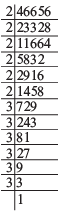Question 2. Find the smallest number by which each of the following numbers must be multiplied to obtain a perfect cube.

(i) 243 (ii) 256 (iii) 72
(iv) 675 (v) 100

Solution:

(i) We have 243 = 3 * 3 * 3 * 3 * 3

The prime factor 3 is not a group of three.

∴ 243 is not a perfect cube.

Now,  * 3 = [3 * 3 * 3 * 3 * 3] * 3

or 729 = 3 * 3 * 3 * 3 * 3 * 3

Now, 729 becomes a perfect cube.

Thus, the smallest required number to multiply 243 to make it a perfect cube is 3.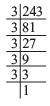(ii) We have 256 = 2 * 2 * 2 * 2 * 2 * 2 * 2 * 2

Grouping the prime factors of 256 in triples, we are left over with 2 * 2.

∴ 256 is not a perfect cube.

Now,  * 2 = [2 * 2 * 2 * 2 * 2 * 2 * 2 * 2] * 2

or 512 = 2 * 2 * 2 * 2 * 2 * 2 * 2 * 2 * 2 i.e. 512 is a perfect cube.

Thus, the required smallest number is 2.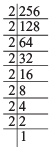(iii) We have 72 = 2 * 2 * 2 * 3 * 3

Grouping the prime factors of 72 in triples, we are left over with 3 * 3.

∴ 72 is not a perfect cube.

Now,  * 3 = [2 * 2 * 2 * 3 * 3] * 3

or 216 = 2 * 2 * 2 * 3 * 3 * 3

i.e. 216 is a perfect cube.

∴ The smallest number required to multiply 72 to make it a perfect cube is 3.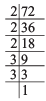(iv) We have 675 = 3 * 3 * 3 * 5 * 5

Grouping the prime factors of 675 to triples, we are left over with 5 * 5.

∴ 675 is not a perfect cube.

Now,  * 5 = [3 * 3 * 3 * 5 * 5] * 5

or 3375 = 3 * 3 * 3 * 5 * 5 * 5

Now, 3375 is a perfect cube.

Thus, the smallest required number to multiply 675 such that the new number is a perfect cube is 5.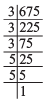(v) We have 100 = 2 * 2 * 5 * 5

The prime factor are not in the groups of triples.

∴ 100 is not a perfect cube.

Now  * 2 * 5 = [2 * 2 * 5 * 5] * 2 * 5

or  * 10 = 2 * 2 * 2 * 5 * 5 * 5

1000 = 2 * 2 * 2 * 5 * 5 * 5

Now, 1000 is a perfect cube.

Thus, the required smallest number is 10.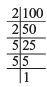Question 3. Find the smallest number by which each of the following numbers must be divided to obtain a perfect cube.

(i) 81 (ii) 128 (iii) 135
(iv) 192 (v) 704

Solution:

(i) We have 81 = 3 * 3 * 3 * 3

Grouping the prime factors of 81 into triples, we are left with 3.

∴ 81 is not a perfect cube.

Now,  /3 = [3 * 3 * 3 * 3] ÷ 3

or  27 = 3 * 3 * 3

i.e. 27 is a prefect cube

Thus, the required smallest number is 3.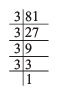(ii) We have 128 = 2 * 2 * 2 * 2 * 2 * 2 * 2

Grouping the prime factors of 128 into triples, we are left with 2.

∴ 128 is not a perfect cube

Now,  /2 = [2 * 2 * 2 * 2 * 2 * 2 * 2] ÷2

or  64 = 2 * 2 * 2 * 2 * 2 * 2

i.e. 64 is a perfect cube.

∴ The smallest required number is 2.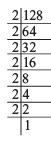(iii) We have 135 = 3 * 3 * 3 * 5

Grouping the prime factors of 135 into triples, we are left over with 5.

∴ 135 is not a perfect cube

Now,  /5 = [3 * 3 * 3 * 5] ÷5

or 27 = 3 * 3 * 3

i.e. 27 is a perfect cube.

Thus, the required smallest number is 5.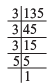(iv) We have 192 = 2 * 2 * 2 * 2 * 2 * 2 * 3

Grouping the prime factors of 192 into triples, 3 is left over.

∴ 192 is not a perfect cube.

Now,  ∏ 3 = [2 * 2 * 2 * 2 * 2 * 2 * 3] ÷3

or  64 = 2 * 2 * 2 * 2 * 2 * 2

i.e. 64 is a perfect cube.

Thus, the required smallest number is 3.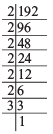(v) We have 704 = 2 * 2 * 2 * 2 * 2 * 2 * 11

Grouping the prime factors of 704 into triples, 11 is left over.

∴  /11 = [2 * 2 * 2 * 2 * 2 * 2 * 11] ÷11

or 64 = 2 * 2 * 2 * 2 * 2 * 2

i.e. 64 is a perfect cube.

Thus, the required smallest number is 11.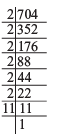Question 4. Parikshit makes a cuboid of plasticine of sides 5 cm, 2 cm, 5 cm. How many such cuboids will he need to form a cube?

Solution: Sides of the cuboid are: 5 cm, 2 cm, 5 cm

∴ Volume of the cuboid = 5 cm * 2 cm * 5 cm

To form it as a cube its dimension should be in the group of triples.

∴ Volume of the required cube = [5 cm * 5 cm * 2 cm] * 5 cm * 2 cm * 2cm = [5 * 5 * 2 cm3] = 20 cm3

Thus, the required number of cuboids = 20

Cube Roots

Finding cube root is the inverse operation of finding cube.

Since, 4= 64, so the cube root of 64 is 4.

The symbol for cube root is ∛

E*amples:

23 = 8 → ∛8 = 2

33 = 27 → ∛27 = 3

4= 64 →∛64 = 4

53 = 125 → ∛125 = 5

63 = 216 → ∛ 216 = 6

73 = 343 → ∛343 = 7

Cube Root of a Cube Number through Estimation

Example 1. Find the cube root of 614125 through estimation.

Solution: We use the following steps to find the cube root through estimation.

I. Form two groups three digits each starting from the right most”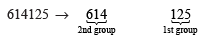II. 1st group (125) gives us the unit’s digit of cube root.

∵ Cube of a number ending in 5, also ends in 5.

∴ Unit’s digit of the cube root is 5.

III. 2nd group (here 614) gives us the ten’s digit of the cube root.

Since, 83 = 512 and 93 = 729

Also 512 < 614 < 729

∴ We guess the ten’s digit of the cube root with the help of unit’s digit of 512.

We know that, if a number ends in 8, its cube will end in 2.

∴ Ten’s digit of the required cube root must be 8.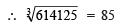Cube Root through Prime Factorisation Method

Example 1. Find the cube root of 1728.

Solution: By prime factorisation, we have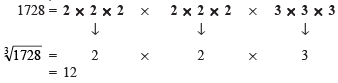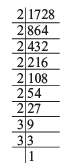Example 2. Find the cube root of 27000 by prime factorisation.

Solution: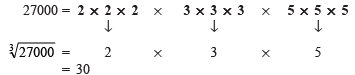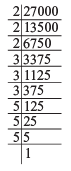Question: State true or false: for any integer m, m2 < m3. Why?

Solution: It is not always true.

For example, let m = –1

We have m2 = (–1)2 = 1

and m3 = (–1)3 = –1

∴ The above statement, i.e. m2 < m3 is false.

## Mathematics (Maths) Class 8

217 videos|147 docs|48 tests

,

,

,

,

,

,

,

,

,

,

,

,

,

,

,

,

,

,

,

,

,

;